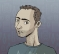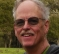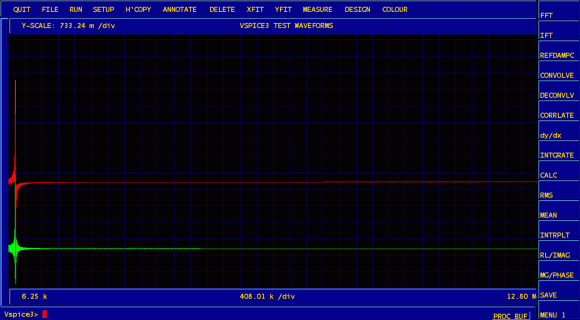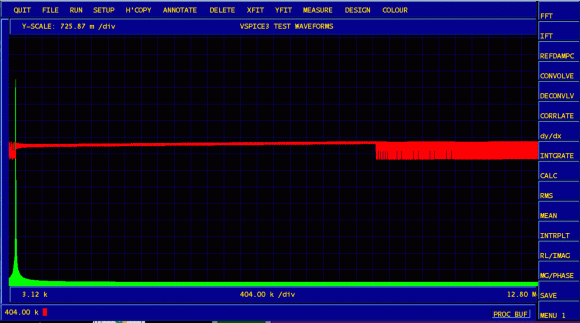## FFT of a sine wave signalStarted by 4 years ago4 replieslatest reply 4 years ago637 views

I am computing FFT of a sine wave signal. Since this is a signal with a single frequency, I was expecting FFT to give one spike indicating the presence of a single frequency but I see 2 frequencies from FFT.

I would appreciate if someone can clarify this ...

Code snippet below:

Fs = 10;            % Sampling frequency

T = 1/Fs;           % Sampling period

L = 200;             % Length of signal

t = (0:L-1)*T;      % Time vector

X = sin(2*pi*t);

Y = fft(X);

P2_raw = abs (Y);

P2 = abs(Y/L);

P1 = P2(1:L/2+1);

P1(2:end-1) = 2*P1(2:end-1);

f = Fs*(0:((L)/2))/(L);

subplot(2,2,1)

plot(t,X)

title('Signal Corrupted with Zero-Mean Random Noise')

xlabel('t (milliseconds)')

ylabel('X(t)');

subplot(2,2,2)

plot(f,P1)

title('Single-Sided Amplitude Spectrum of X(t)')

xlabel('f (Hz)')

ylabel('|P1(f)|');

subplot(2,2,3)

plot(t,P2_raw)

title('P2_raw X(t)')

xlabel('f (Hz)')

ylabel('|P1(f)|');

[ - ]You plot in the third plot time versus P2_raw. It should be:

subplot(2,2,3)

plot(f,P2_raw(1:L/2+1));

[ - ]Well, first of all bertramaerts is right about this part. Similar to what you do in the second subplot, in the third subplot you should plot only (the first) half of your signal.

In general, if this is what you don't understand, when you "Fourier-transform" a real valued signal you will end up with a spectrum which is mirrored by the Fs/2 point (there's more into that, but I wouldn't like to create any confusion here). I am not sure this is what you are asking for, but if it is, you should look a bit more into the mathematics of Fourier transform. If I am not mistaken you can find some good information on this site, but there are also a plethora of good reference textbooks you could consult. Let me (or any other person here) know if you would like to get some recommendations.

Best,

Achilles.

[ - ]Per Achilles comment, the FFT is always complex, even if you feed it a purely real signal. When you combine that with the understanding that a complex signal that has a zero valued complex (quadrature) component is the composition of two signals :

$$(\cos(x) + j\sin(x)) + (\cos(x) - j\sin(x))$$

That is the cosine of the frequency summed with its complex conjugate. So when you look at the output of the FFT you will see both peaks.

Plot only up to the nyquist frequency $$F_{s}/2$$ and you will see the one peak that you expect.

--Chuck

[ - ]